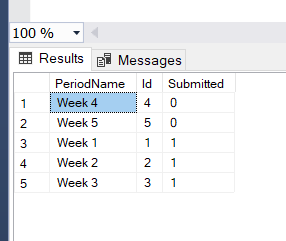# Tricky selects in the same table

Hi all,
I need to make a select on a table with a Bit field called 'Submitted' = true and ordered by Int 'OrderNo' desc. But I also need to select the top1 row in that table when 'Submitted' = false and ordered by OrderNo asc.
I need these in the one select with the 1 unsubmitted row as being the first row of the rows returned.
I can do them separately but am running into problems on how to do this in the 1 select.

Thanks!

``````SELECT
x.OrderNo, x.other_col1, x.other_col2 --, ...
FROM (
SELECT 1 AS sort_seq, *, ROW_NUMBER() OVER(ORDER BY OrderNo) AS row_num
FROM dbo.a_table
WHERE submitted = 'false' /*= 0*/
UNION ALL
SELECT 2 AS sort_seq, *, ROW_NUMBER() OVER(ORDER BY OrderNo DESC) AS row_num
FROM dbo.a_table
WHERE submitted = 'true' /*= 1*/
) AS x
ORDER BY x.sort_seq``````

I tried that but the data returned is slightly off - I tried a few changes with it but cant get it.

The below is my query and a shot of the data returned. It should not be returning Week 5 as it should only return 1 unsubmitted and the order of the submitted should be desc. It appears the asc and desc in the 2nd part of query make no difference?

Again, thanks for any direction.

``````SELECT
x.PeriodName, x.Id, x.Submitted
FROM (
SELECT 1 AS sort_seq, *, ROW_NUMBER() OVER(ORDER BY OrderNo ) AS row_num
FROM dbo.lk_MFPeriod
WHERE Submitted = 'false' /*= 0*/
UNION ALL
SELECT 2 AS sort_seq, *, ROW_NUMBER() OVER(ORDER BY OrderNo DESC) AS row_num
FROM dbo.lk_MFPeriod
WHERE Submitted = 'true' /*= 1*/
) AS x
ORDER BY x.sort_seq
``````please provide DDL for lk_MFPeriod and sample data using DML

Sorry, I slightly misunderstood the requirement. Try this adjusted query:

``````SELECT
x.OrderNo, x.other_col1, x.other_col2 --, ...
FROM (
SELECT TOP (1) 1 AS sort_seq,  --<<--<<-- added TOP (1)
*, ROW_NUMBER() OVER(ORDER BY OrderNo) AS row_num
FROM dbo.a_table
WHERE submitted = 'false' /*= 0*/
UNION ALL
SELECT 2 AS sort_seq, *, ROW_NUMBER() OVER(ORDER BY OrderNo DESC) AS row_num
FROM dbo.a_table
WHERE submitted = 'true' /*= 1*/
) AS x
ORDER BY x.sort_seq, OrderNo /*DESC*/``````
1 Like

Thanks a million Scott - that solved it!

For others - I also just uncommented Scott's last DESC at last line and its working perfectly!

Much appreciated!

``````SELECT
x.PeriodName, x.Id, x.Submitted
FROM (
SELECT TOP (1) 1 AS sort_seq,  --<<--<<-- added TOP (1)
*, ROW_NUMBER() OVER(ORDER BY OrderNo) AS row_num
FROM dbo.lk_MFPeriod
WHERE submitted = 'false' /*= 0*/
UNION ALL
SELECT 2 AS sort_seq, *, ROW_NUMBER() OVER(ORDER BY OrderNo DESC) AS row_num
FROM dbo.lk_MFPeriod
WHERE submitted = 'true' /*= 1*/
) AS x
ORDER BY x.sort_seq, OrderNo DESC``````